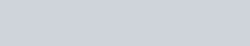Use Lewis symbols to show electron transfer between the following atoms to form cations and anions
Question:

Use Lewis symbols to show electron transfer between the following atoms to form cations and anions

(a) K and S

(b) Ca and O

(c) Al and N.

Solution:

(a) K and S:

The electronic configurations of K and S are as follows:

K: 2, 8, 8, 1

S: 2, 8, 6Sulphur (S) requires 2 more electrons to complete its octet. Potassium (K) requires one electron more than the nearest noble gas i.e., Argon. Hence, the electron transfer can be shown as:(b) Ca and O:

The electronic configurations of Ca and O are as follows:

Ca: 2, 8, 8, 2

O: 2, 6

Oxygen requires two electrons more to complete its octet, whereas calcium has two electrons more than the nearest noble gas i.e., Argon. Hence, the electron transfer takes place as:(c) Al and N:

The electronic configurations of Al and N are as follows:

Al: 2, 8, 3

N: 2, 5

Nitrogen is three electrons short of the nearest noble gas (Neon), whereas aluminium has three electrons more than Neon. Hence, the electron transference can be shown as: